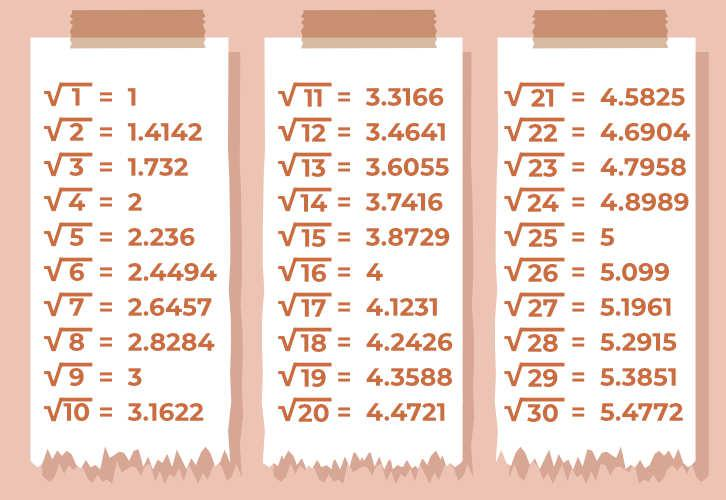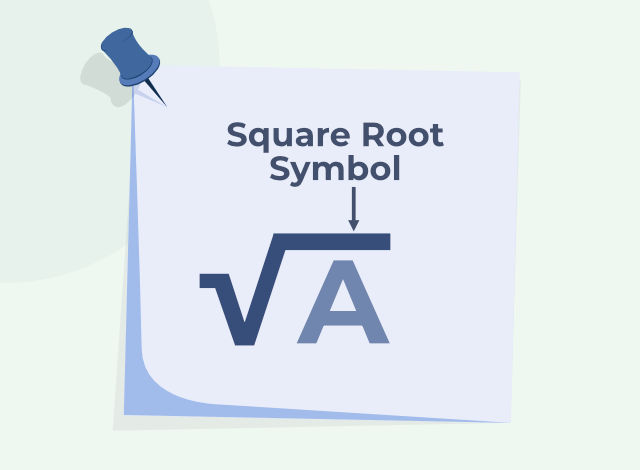GFG App
Open AppBrowser
Continue

Square root symbol or square root sign is denoted by the symbol ‘’. It is a mathematical symbol used to represent square roots in mathematics. The square root symbol (√) is also called Radical. For example, we write the square root of 4 as √(4). It is read as root 4 or the square root of 4.

## What is Square Root?

A square root is a number that gives the original number when multiplied by the given number itself. The square root is represented by the symbol.

Let’s consider the number “A” which is a positive integer, such that √(A×A) = √(A2) = A

The image showing the square root of the first 30 natural numbers is,Example: Find the square root of 36

Solution:

√(36)= √(6×6) = 6

Square root of 36 is 6

### Concept of Square Root

The concept of a square root can be explained using the following steps:

Step 2: Divide the radicand by any perfect square factor until there are no more perfect square factors left.

Step 3: Write the remaining factors under the radical symbol, and simplify if possible.

## Square Root Symbol

The square root of any number is represented using the symbol “” i.e. square root of 1 is represented as √(1), the square root of 25 is represented as √(25) and similarly, the square root of other numbers can easily be represented.

The image showing the square roots symbol is,The other name given to the square root symbol is radical. Some mathematicians also called it Surds.

## Simplifying Square Roots

This involves simplifying a square root by finding perfect square factors of the radicand and writing them outside the radical symbol.

Example: Simplify √50.

Solution:

√50 = √(25 × 2)

= √(5 × 5 × 2)

= 5√2

### Rationalizing the Denominator

This involves multiplying the numerator and denominator of a fraction by the conjugate of the denominator to eliminate the radical from the denominator.

Example: Rationalize the denominator of 1/√5.

Solution:

Multiply the numerator and denominator by √5 to get (1 x √5)/(√5 x √5) = √5/5.

### Using Imaginary Numbers

This involves using the imaginary unit “i”, which is defined as the square root of -1, to represent numbers that cannot be expressed as real numbers.

Example: Find the square root of -25.

Solution:

√(-25) = √(5 × 5 × -1) = 5i

### Repeated Subtraction Method

Subtracting the consecutive odd numbers from the given number till the difference is zero and the required square root is the number of times we subtracted the given number.

Example: Square root of 36

Solution:

• 36-1 = 35
• 35-3 = 32
• 32-5 = 27
• 27-7 = 20
• 20-9 = 11
• 11-11 = 0

Here the number is subtracted 6 times. Hence the square root of 36 is 6

## Perfect Squares from 1 to 100

Perfect squares from 1 to 100 are discussed in the table

## Square of First 20 Natural Numbers

The square of the first 20 natural numbers is discussed below in the table,

## Square Root of First 20 Natural Numbers

The square root of the first 20 natural numbers is discussed below in the table,

Also, Check

## Solved Examples on Square Roots

Example 1: Estimate the square root of 72.

Solution:

The perfect squares closest to 72 are 64 and 81.

The square root of 64 is 8, and the square root of 81 is 9.

Therefore, the square root of 72 is estimated to be between 8 and 9.

Example 2: Simplify √27.

Solution:

We can factor 27 as √(9 × 3), and since the square root of 9 is 3, we can simplify it as 3√3.

Example 3: Simplify √75.

Solution:

We can factor 75 as √(25 × 3), and since the square root of 25 is 5, we can simplify it as 5√3.

Example 4: Simplify 4 / (√2 + √3)

Solution:

To rationalize the denominator, we multiply both the numerator and denominator by (√2 – √3).

= 4×(√2 – √3)/(√2 + √3)(√2 – √3)

= 4×(√2 – √3)/(√2x√2 – √3 √3)

= 4×(√2 – √3)/(2-3)

This gives us [4(√2 – √3)] / (-1), which simplifies to -4(√2 – √3)

Example 5: Simplify (3 + √5) / (√5 – 1)

Solution:

To rationalize the denominator, we multiply both the numerator and denominator by (√5 + 1).

= (3 + √5)(√5 + 1) / (√5 – 1)(√5 + 1) (multiplying by the conjugate of the denominator)

= (3√5 + 3 + √5√5 + √5) / (5 – 1) (expanding the numerator and denominator)

= (4√5 + 8) / 4

= 4(2 + √5) / 4 (cancelling numerator and denominator)

= 2+√5

This gives us [(3 + √5)(√5 + 1)] / (5 – 1), which simplifies to 2 + √5

Example 6: Find the square root of -16.

Solution:

Since the square root of -16 is not a real number,

We can represent it as a complex number of the form a + bi. In this case, we have a = 0 and b = 4.

Therefore, the square root of

-16 = √(i2(4)2)

= 4i

Example 7: Find the square root of -3 – 4i.

Solution:

To find the square root of a complex number we can use the formula,

√(a + bi) = ±(√[(a + √(a2 + b2))/2] + i√[(|a – √(a2 + b2)|)/2])

Applying this formula to the complex number -3 – 4i, we have a = -3 and b = -4. Therefore, we can substitute these values into the formula,

√(-3 – 4i) = ±(√[(-3 + √(9 + 16))/2] + i√[(|-3 – √(9 + 16)|)/2])

= ±(√[(-3 + √(25))/2] + i√[(|-3 – √(25)|)/2])

= ±(√[(-3 + 5)/2] + i√[(|-3 – 5|)/2])

= ±(√(2/2) + i√(|-8|/2))

= ±(√(2/2) + i√(8/2))

= ±(√1 + i√4)

= ±(1 + 2i)

Example 8: Simplify 4 / (√2 – √3)

Solution:

To rationalize the denominator, we multiply both the numerator and denominator by (√2 + √3).

= 4×(√2 + √3)/(√2 – √3)(√2 + √3)

= 4×(√2 + √3)/(√2x√2 – √3 √3)

= 4×(√2 + √3)/(2-3)

This gives us [4(√2 + √3)] / (-1), which simplifies to -4(√2 + √3)

## FAQs on Square Roots

### Q1: What is the square root of a number give one example.

A square root is a number that gives the original number when multiplied by the given numberitself.

Example: Find the square root of 36

√(49)= √(7×7) = 7

The square root of 49 is 7

### Q2: Give the symbol for representing the square root and the name of that symbol.

The square root can be represented by using the symbol “√” and we can call it a radical symbol

### Q3: What is the difference between a radical and a square root?

A radical is a mathematical symbol that represents a root, while a square root specifically refers to the root of a number that is multiplied by itself.